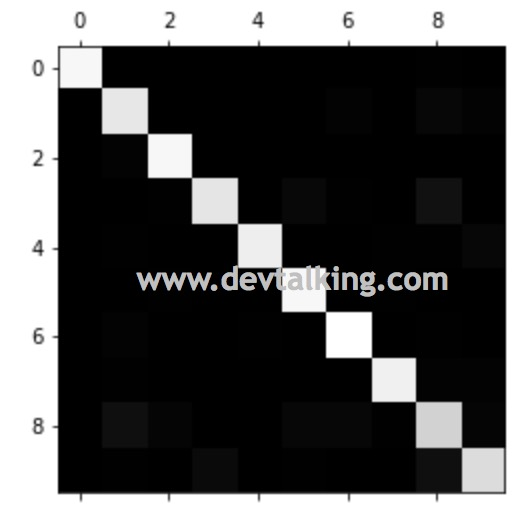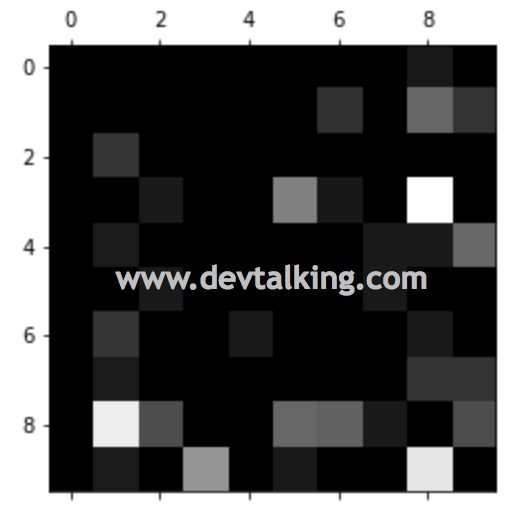混淆矩阵（Confusion Matrix）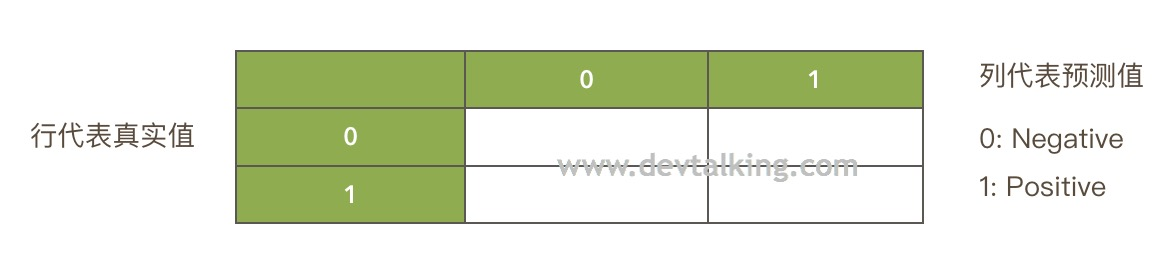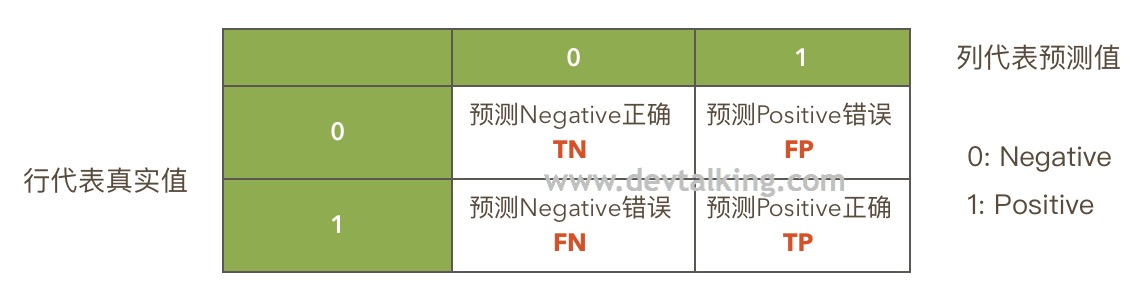• (0, 0)格子真实值和预测值都为0，称为预测Negative正确，记作True Negative，简写为TN。
• (0, 1)格子真实值为0，但预测值为1，称为预测Positive错误，记作False Positive，简写为FP。
• (1, 0)格子真实值为1， 但预测值为0，称为预测Negative错误，记作False Negative，简写为FN。
• (1, 1)格子真实值和预测值都为1，称为预测Positive正确，记作True Positive，简写为TP。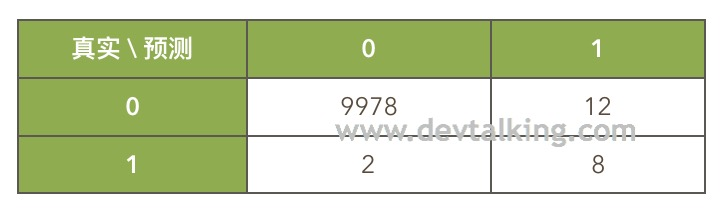• 没有患癌症，系统也预测出没有患癌症的人为9978人（TN）。
• 没有患癌症，但系统预测出患癌症的人为12人（FP）。
• 患有癌症，但系统预测出没有患癌症的人为2人（FN）。
• 患有癌症，系统也预测出患有癌症的人为8人（TP）。

精准率（Presicion）

$$precision = \frac {TP} {TP + FP}$$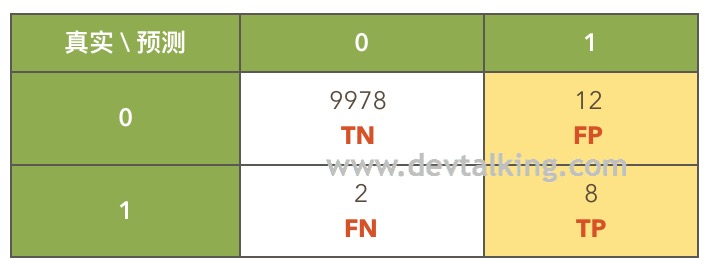召回率（Recall）

$$recall = \frac {TP} {TP + FN}$$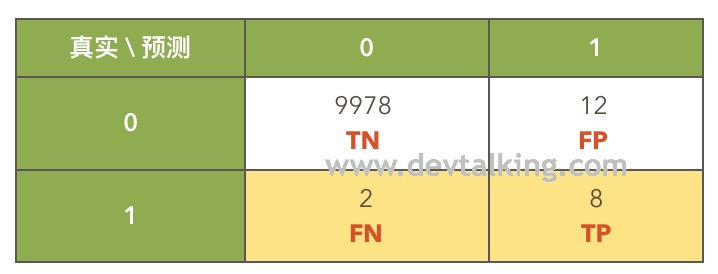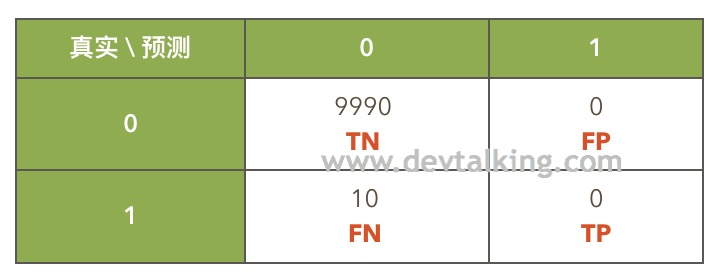• 分类准确度：9990 / 10000 = 99.9%
• 精准率：0 / (0+0)，无意义，既为0
• 召回率：0 / (10 +0) = 0

实现精准率和召回率

• y被拆分后的测试数据量y_test为450，混淆矩阵中的四个熟总和为450。
• 真值为0，但预测值为1有2个。
• 真值为1，但预测值为0有9个。
• 真值为1，预测值也为1有36个。

F1 Score

F1 Score定义

$$\frac 1 {F1} = \frac 1 2 (\frac 1 {precision} + \frac 1 {recall})$$

$$F1 = \frac {2 \cdot precision \cdot recall} {precision + recall}$$

Scikit Learn 中的 F1 Score

Scikit Learn中也封装了F1 Score，但是它封装时传的参数和我们实现的不太一样，它只需要传入真值和预测值既可，精准率和召回率是在函数中计算的：

精准率和召回率直接的平衡

$$\theta^T \cdot X_b = 0$$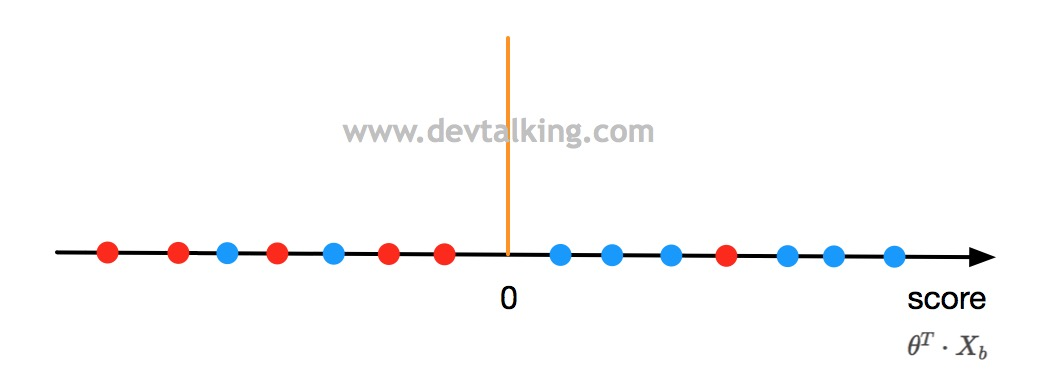$$\theta^T \cdot X_b = threshold$$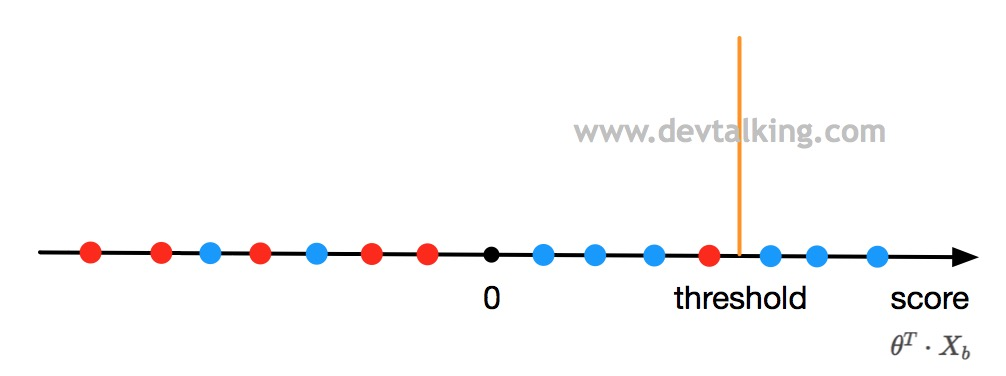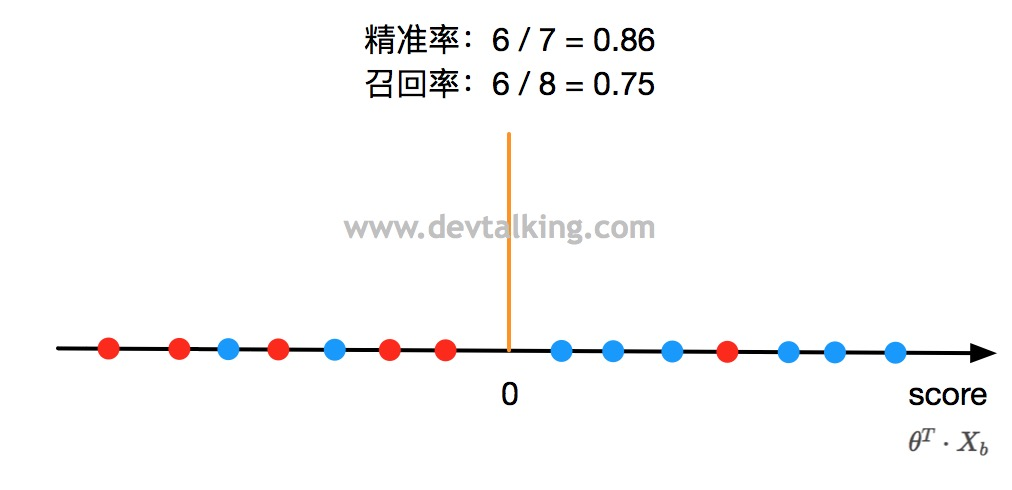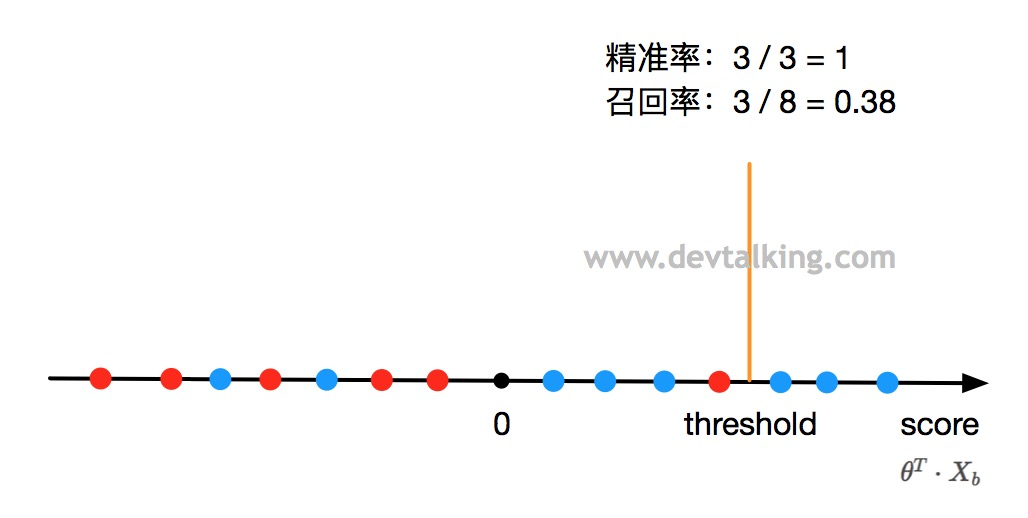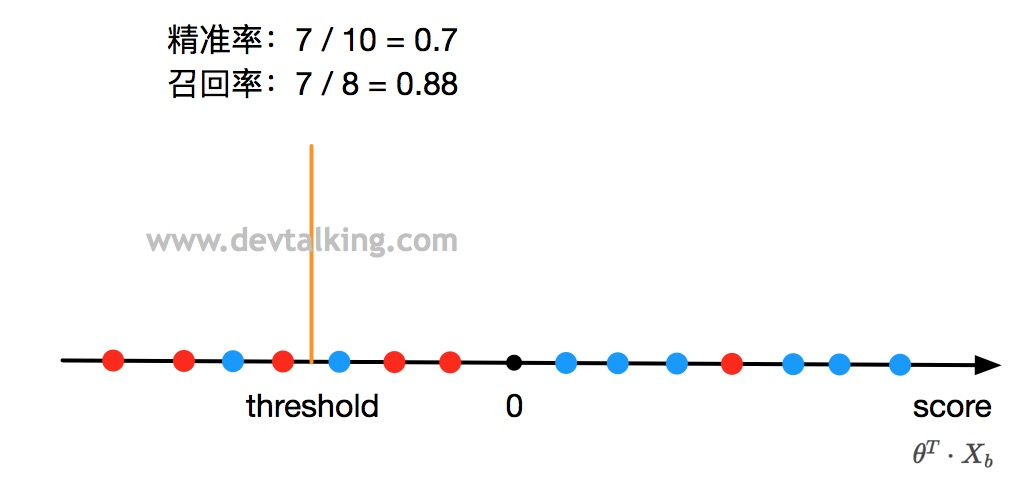通过程序验证精准率和召回率的平衡关系

Scikit Learn中的confusion_matrixprecision_scorerecall_score函数都是基于$threshold$为0计算的，也就是判断decision_score中的所有值，如果大于0就分类为1，如果小于0就分类为0。那我们现在将$threshold$调大一点，比如将5作为区分1和0的分界点，那么我们的预测值就可以这样求：

PR曲线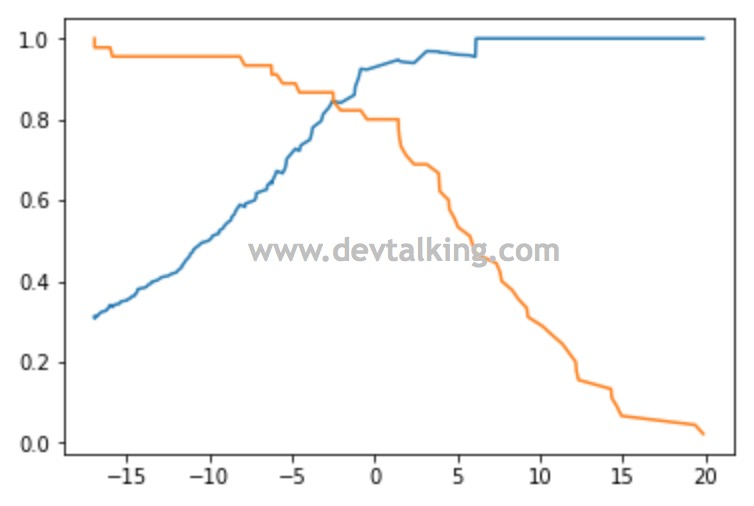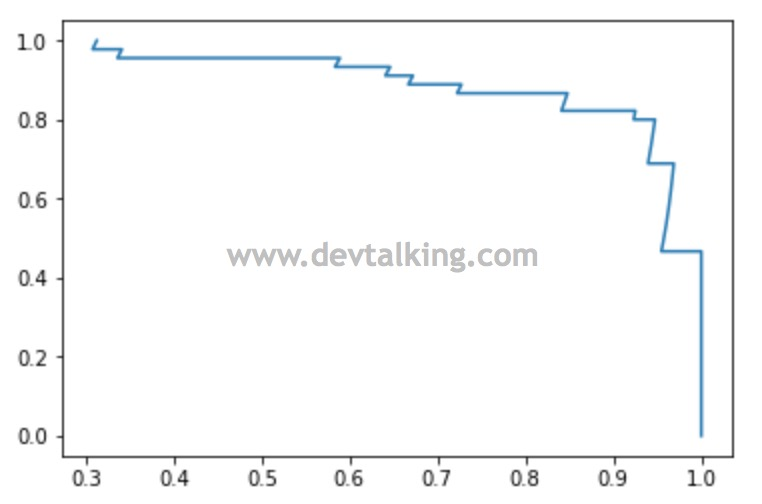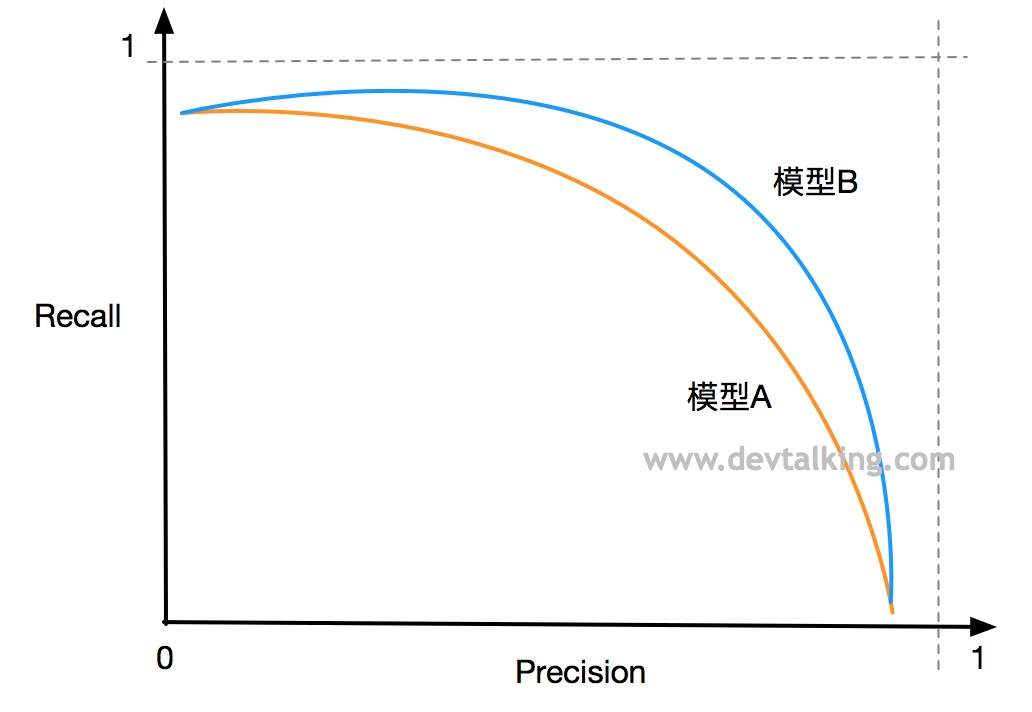ROC曲线

FPR和TPR

FPR和TPR同样是基于混淆矩阵而来的，FPR的公式为：

$$FPR=\frac {FP} {TN + FP}$$

TPR的公式为：

$$TPR = \frac {TP} {TP + FN}$$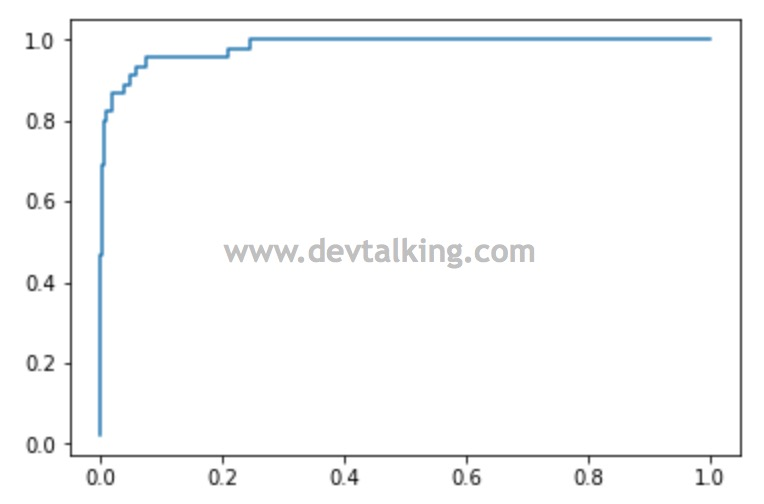ROC曲线和PR曲线有一个不同之处是，ROC曲线对极度有偏的数据是不敏感的。所以如果样本数据有极度有偏的情况时，通常还是主要使用PR曲线来判断模型的好坏，ROC曲线辅助判断。

多分类问题中的混淆矩阵

Scikit Learn 的precision_score方法有一个average参数，默认值为binary，既默认计算二分类问题。如果要计算多分类问题，需要将average参数设置为micro